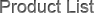# Heat Consumption and Production of Grain Dryer

1. Computer SimulationA common problem in using grain dryer is the original moisture of the grain is changeable. For meet the requirement of final moisture, the grain flow (capacity) should be adjusted constantly. We should know the dryer capacity for comparing the dryer performance and it has to be converted. We regard Computer Simulation Methods as a good and feasible way to convert heat rate and capacity, that is, establish a mathematical model of grain drying process, compile a drying simulation program, analog calculate in computer and get the convertion coefficient at last.

The advantage of this way is universal, which can calculate the drying performance and convertion coefficient of different models (dryers of downstream, countercurrent, transverse flow and mixed flow) and different grains (corns, wheats and rice). It can convert every drying condition with fast computation speed; and it can be used to all kinds of drying technology.

The disadvantage is that the analogy method is not universal enough, it needs a certain basis of computer engineering to master the method, and the users have no the software. In addition, mathematical model of the drying process is not accurate enough. So it should improve the research and analogy method, calculation steps is as follows:

• Establish drying process model;
• Develop various kinds of computer simulation program of grain drying technology;
• Calculate heat rate and capacity of the dryer in computer standard conditions by using of simulation program;
• Analog calculate heat rate and capacity in non-standard conditions;
• Calculate convertion coefficient of heat rate and capacity;
• Convert dryer performance.

2.ISO11520一2 International Standard Law

ISO(Intemational Standard Oganization), international drying equipment performance test provides a convertion method, it converts the moisture evaporativity get from the test with four correction coefficient of K1、K2、K3、K4. Here is the meaning of each correction coefficient:

K1——moisture correction coefficient, K1=(8.971一0.05578Td)X.+1.139InTd一4.652.
K2——hot air temperature correction coefficient, K2=(0.00565-0.000061Td)+0.000915Td+0.915.
K3——air humidity correction coefficient, K3=1.0175一0.01072(l一Φ).
K4——blowing rate correction coefficient, K4=(0.022Td一3.445)a/V一0.271InTd+2.608.

3. Data Sheet Method

List a table by heat calculation with convertion coefficient of parameter variation in all conditions, then convert with interpolation method, such as the method used in Russia. The standard provides two kinds of tables, one is atmospheric conditions convertion table, another is grain condition convertion table, find the two coefficients from the table, and their product is the total convertion coefficient.

In the basis of deep analysis and study of existing research result domestic and overseas, this article analyses and discusses the convertion standard condition, provides the calculating method and service conditions of the conversion coefficient of each dryer model and each grain drying technology.